# High School Math : The Unit Circle and Radians

## Example Questions

← Previous 1 3 4 5 6

### Example Question #1 : Using The Unit Circle

What is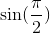?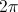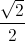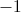Explanation:

If you examine the unit circle, you'll see that the the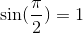. If you were to graph a sine function, you would also see that it crosses through the point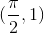.

### Example Question #2 : Using The Unit Circle

What is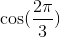?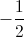Explanation:

If you look at the unit circle, you'll see that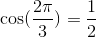. You can also think of this as the cosine of, which is also.

### Example Question #3 : The Unit Circle And Radians

What is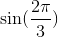?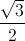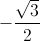Explanation:

If you look at the unit circle, you'll see that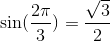. You can also think of this as the sine of, which is also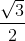.

### Example Question #4 : The Unit Circle And Radians

What is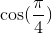?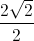Explanation:

Using the unit circle,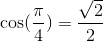. You can also think of this as the cosine of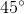, which would also be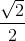.

### Example Question #3 : Using The Unit Circle

What is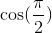?Explanation:

Using the unit circle, you can see that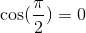. If you were to graph a cosine function, you would also see that it crosses through the point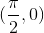.

### Example Question #6 : The Unit Circle And Radians

What is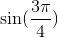?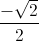Explanation:

Using the unit circle,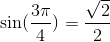. You can also think of this as the sine of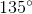, which would also be.

### Example Question #4 : Using The Unit Circle

What is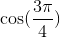?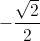Explanation:

Using the unit circle, you can see that the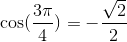. Since the angle is in Qudrant II, sine is positive and cosine is negative.

### Example Question #8 : The Unit Circle And Radians

What is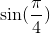?Explanation:

Using the unit circle,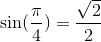. You can also think of this as the sine of, which would also be.

### Example Question #72 : Pre Calculus

What is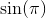?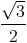Explanation:

If you examine the unit circle, you'll see that that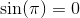### Example Question #10 : The Unit Circle And Radians

What is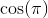?If you examine the unit circle, you'll see that the value of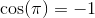. You can also get this by examining a cosine graph and you'll see it crosses the point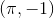.